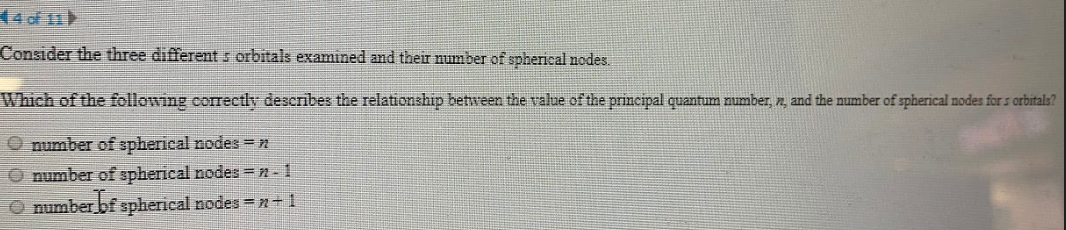# Consider the three different s oritals examined and their number of spherical nodes. Which of the following correctly describes the relationship between the value of the principal quantum number, n, and the number of spherical nodes for s orbitals. a) number of spherical nodes = n b) number of spherical nodes = n - 1 c) number of spherical nodes = n + 1